Simulation of Kerr Lens Modelocking Behavior in Sagittal Plane | OMICS International
Journal of Lasers, Optics & Photonics

# Simulation of Kerr Lens Modelocking Behavior in Sagittal Plane

Kamjoo MJ*

Basic science Department, Physics group, Imam Hossein University, Tehran, Iran

*Corresponding Author:
Kamjoo MJ
Basic science Department, Physics group
Imam Hossein University, Tehran, Iran
Tel: +98 21 7281 8142
E-mail: [email protected]

Received Date: October 07, 2016; Accepted Date: December 27, 2016; Published Date: January 10, 2017

Citation: Kamjoo MJ (2017) Simulation of Kerr Lens Modelocking Behavior in Sagittal Plane. J Laser Opt Photonics 4: 146. doi: 10.4172/2469-410X.1000146

Copyright: © 2017 Kamjoo MJ. This is an open-access article distributed under the terms of the Creative Commons Attribution License, which permits unrestricted use, distribution, and reproduction in any medium, provided the original author and source are credited.

Visit for more related articles at Journal of Lasers, Optics & Photonics

#### Abstract

Femtosecond oscillator is first step to generate femtosecond laser pulse. Simulation of the laser beam behavior in the oscillator cavity, especially in the gain medium is essential to realize and optimize the oscillator output pulse characteristics. In this work, at first we simulate the laser beam propagation in a folded cavity with curved mirrors in symmetric and asymmetric designs using the ABCD ray tracing technique for a Gaussian beam shape to achieve influence of Kerr lens modelocking in sagittal plane. We use split-step method to obtain the beam intensity changes in the ti:sa rod and shooting method to calculate the beam spot size on the first cavity mirror. In following the influence of the gain medium position changes on the size and position of the beam waist and also on the misalignment sensitivity parameter for the sagittal plane is simulated. Furthermore the misalignment sensitivity parameter and the size and position of the beam waist are not identical for the beams in the sagittal plane, therefore to actualize an optimum design it is necessary to simulate the beam propagation in plane which is not usually considered in early design because of complication of the simulation.

#### Keywords

Laser beam; Refractive index; Electric field; Kerr lens

#### OCIS codes

(320.7110) Ultrafast nonlinear optics, (190.3270) Kerr effect

#### Introduction

Femtosecond pulse laser is one of the most applied lasers and its oscillator is footstone in femtosecond pulse generation. Modelocking is a straightforward method to generate femtosecond pulses and Kerr lens effect is a widespread way to lock the oscillator cavity modes. The Kerr effect induced self-focusing [1,2] during an intense pulse passing through a gain medium along focal range is exploited for modelocking . The optical Kerr effect causes a refractive index intensity dependence which induces lensing in the gain medium. The beam profile changes inside the oscillator cavity by the Kerr effect. Several investigations have been carried out about the Kerr lens modelocking [4-8]. The Kerr lensing causes the intense pulsed radiation inside the cavity gets narrower diameter and tighter focus than the CW radiation. Experimentally, when a suitable aperture is located inside the cavity, main part of the CW radiation will be eliminated by the aperture and therefore receives higher losses than the pulsed radiation. The combination of the Kerr lens-aperture acts as a fast saturable absorber and a fast passive gain modulation is obtained. At the beginning of the Kerr modelocking developments, intensity fluctuations in laser startup were not sufficient to induces strong and steady Kerr lens modelocking [9-10] so acousto-optic modulation, additive pulse modelocking, impulsive starting or other methods [11-21] was required before the Kerr lens effect modelocking. Self-starting Kerr lens modelocking was obtained by use of highly nonlinear Kerr effect gain medium. A selfstarting femtosecond oscillator exploiting Kerr lens modelocking was introduced by Sibbet et al. .

In this paper, we first simulate the laser beam propagation in a folded cavity with curved mirrors in symmetric and asymmetric designs using the ABCD ray tracing technique for a Gaussian beam shape to achieve influence of Kerr lens modelocking in sagittal plane. We use split-step method to obtain the beam intensity changes in the tisa rod as the gain medium and shooting method to calculate the beam spot size on the first cavity mirror.

In previous works the influence of the Kerr lensing during the laser beam propagation were simulated [3,23] but the astigmatism due to the cavity curved mirrors and the nonlinear Kerr medium were assumed negligible and not considered in the computation due to complication of simulation [24,25]. In this paper, for more precise beam propagation analysis inside the oscillator, the astigmatism is considered and the laser beam propagation in the sagittal plane is separately simulated. The changes in the beam waist size and its position also the oscillator misalignment sensitivity parameter in the sagittal plane are illustrated.

#### Beam Propagation in Oscillator

An oscillator with four-mirror cavity is considered in this simulation and shown in the Figure 1. The folded cavity setup changes the beam propagation behavior and displaces the focus points in the sagittal plane. Without the Kerr lens effect consideration, the astigmatism can be minimized by using the gain medium cut in its Brewster angle and rotating the cavity curved mirrors in a special angle . Considering the Kerr lensing, the calculation of curved mirrors angle will not be valid for astigmatism compensation and the beam propagation in sagittal plane should not be neglected. The ABCD matrix is used to determine the beam profile in the oscillator .

Ray tracing

The concept of ABCD matrix for a beam propagation is shown in Figure 2 Ray tracing of a beam through series of optical components is in the form of multiplication of 2*2 ABCD matrices.

Figure 2: Beam propagates through an optical component, it enters in position ri and exit from it at ro.

When a beam propagates through an optical component, it enters in position ri with slope of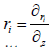and exit from it at r0 with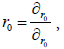respectively. This can be written in form of an ABCD matrix.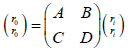(1)

For one round trip in oscillator we have total ABCD matrix as: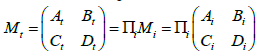(2)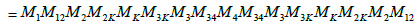The required ABCD matrices for beam propagation simulation inside the oscillator are shown in Table 1. The resonator stability condition is satisfied by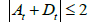that gives the allowed displacement range between the cavity curved mirrors (l23=l2k+lB+l3k). The oscillator misalignment sensitivity parameter in the stability region is determined by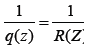where larger Ct implies lower sensitivity of the oscillator to misalignment .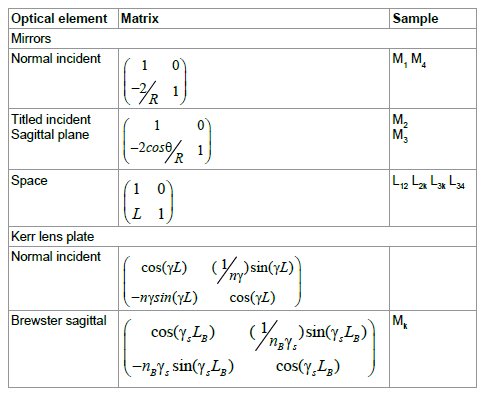Table 1: Matrices of elements used in the simulation.

Fundamental transverse mode of a Gaussian beam in an oscillator is given by :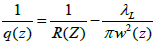(3)

Where R(z) and W(z) are the beam wave front radius of curvature and the beam spot radius at position z, respectively and λL is the beam wavelength.

The laser beam size is defined by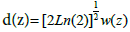. The beam parameter q(z) among propagation from z1 to z2 can be written as: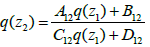(4)

From the stability conditions in the cavity the beam wave front radius of curvature has to be equal to the mirror radius . In the oscillator the input plane mirror M1 is located at z=0 with the radius R1=∞, therefore q parameter after one roundtrip on this mirror is given by: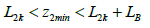(5)

With consideration of the Kerr lens effect, the ABCD elements of one roundtrip matrix will depend on the laser beam radius (W(z)). Now shooting method is applied to calculate the beam size on the first mirror. In this method, first an arbitrary beam radius is chosen to start ray tracing for one roundtrip. If the chosen radius is correct, the equality equation (Eq. 7) must be fulfilled. Otherwise another radius must be chosen and repeat the calculation to obtain beam radius on the first mirror.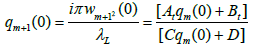(6)

In the Eq.6 the index m stands for the number of the chosen arbitrary radius.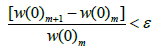(7)

Where ε is the precision limit of the calculation. (Here it is assumed to be 10-5).

For calculation of the intensity changes inside the Kerr lens medium, the crystal is splitted to thin layers and the beam propagation within each thin layer is calculated. The reason for this method is intensity dependency of the each elements of the overall medium matrix along the crystal. Intensity inside each thin layer is different than the others, but inside each layer is assumed to be constant. Each thin layer has its own intensity dependent ABCD matrix which is used as initial matrix to calculate the beam size in the next thin layer of the crystal. In the same way the beam size at the output face of the crystal is obtained. The combination matrix of all thin layer matrices simulates the medium matrix.

Kerr effect on beam propagation

When intensity of an input beam to a nonlinear medium is higher than a threshold, the medium response will be nonlinear and intensity dependent. In this regime the nonlinear refractive index is given by :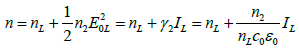(8)

Where n2 and γ2 are the nonlinear refractive index and nonlinear coefficient of the medium, respectively. The electric field amplitude, intensity of the beam and linear refractive index of the medium are shown by E0L, IL and nL, respectively. ε0 and c0 are electric permittivity and light speed in the free space, respectively. Transverse distribution of the fundamental mode of a Gaussian beam can be approximated by Taylor expansion as: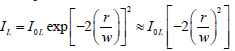(9)

Therefore the refractive index spatial distribution can be rewritten as :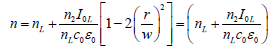(10)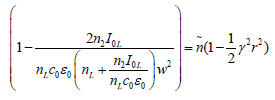Where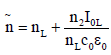and γ is given by: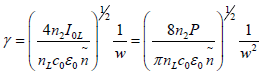(11)

P stands for the laser beam power given by: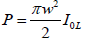(12)

The Kerr lens effect in the Brewster plate is different in sagittal plane. Therefore it is essential that in the Eqs. 10-12, the beam waist and the intensity are replaced as, w→ws = w and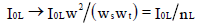, respectively. For the sagittal plane in the Brewster plate we obtain (Table 2):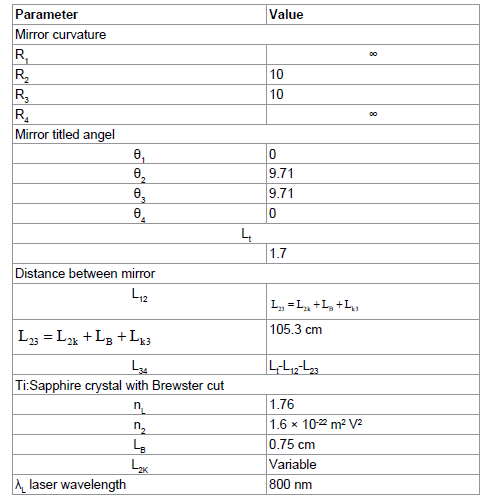Table 2: Oscillator parameter used in simulation.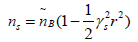(13)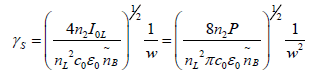Where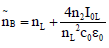for a plane. The Kerr lens effect matrix elements for the sagittal plane in the crystal with length of LB are indicated in the Table 1.

#### Simulation and Results

When an enough powerful beam propagates inside a nonlinear medium showed in Figure 1 the Kerr lensing rises in the medium. To show difference of the Kerr effect on the beam propagation in sagittal plane the distance between the crystal and mirror M2 is considered as a variable (L2k). The implemented parameters for the ti:sa rod simulation are indicated in the Table 2.

In the achieved results, 1/Ct is the optical misalignment sensitivity parameter, dmin and z2min are the beam waist size and its distance to the mirror M2, respectively. The beam waist size is given as: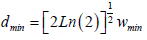(14)

With considering the effect of the Kerr lens, the matrix elements will depend on the beam waist. Therefore shooting method is used to obtain the beam waist size on the mirror M1. After finding the beam waist on the first mirror, W01, the beam waist on the input face of the gain medium crystal is extracted. Then the split-step method is employed to get the total matrix of the crystal (Figures 3-6).

Figure 3: The beam waist distance from M (z2min) vs. the crystal distance from M2 (L2k) for the sagittal ray.

Figure 4: 1/Ct parameter for a round trip Matrix vs. the crystal distance from M2 (L2k) for the sagittal ray.

Figure 5: Beam size in length of crystal (z) vs. the crystal distance from M2 (L2k) for a) the sagittal.

Figure 6:Beam waist size inside the crystal vs. the crystal distance from M2 (L2k) for the sagittal and ray.

Having the oscillator roundtrip matrix and the beam size on the first mirror, the beam size and curvature at any point inside the oscillator can be calculated.

By changing the crystal position to obtain the size and position of the beam waist in the cavity two considerations must be regarded. First, when the beam waist is located outside of the crystal, the transfer equations and transfer matrices have to be employed. Second, when the beam waist is located within the crystal, the minimum beam size and its location in split step method must be considered as the beam waist and its location in the crystal, respectively. As shown in the Figure 3, by increasing the distance of the crystal from the mirror M2, as the beam waist remains outside of the crystal its position stays almost constant. When the beam waist arrives in the crystal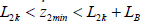the beam waist distance from M2 starts a fast decreasing until the beam waist leaves the crystal. This happens because of increasing the Kerr lens effect due to the increase of the beam intensity inside the crystal. By continuing the crystal movement from the M2 the beam waist position again remains almost constant. Kerr lensing effect is more intensive in the vicinity of L2k=z2min-LB and L2k=z2min in (at Brewster plate) because of high intensity of the beam .

The optical misalignment sensitivity parameter (1/Ct) is shown in Figure 4 for the sagittal ray. For minimizing the misalignment sensitivity parameters 1/Ct must approach zero.

The position and size of the beam waist is critical for the soft and hard aperture Kerr lens mode locking [5,19,32]. Figures 5 and 6 show the beam size as function of L2k inside the crystal and the beam waist size in the cavity as L2k, respectively for the sagittal and the tangential rays. It is demonstrated that the beam sizes in the sagittal plane are not identical and also the beam positions in the same way. Hence for a precise simulation and cavity design, the beam size and its position in the sagittal plane has to be regarded. Optimum condition reveals as a compromise between the beam propagation in sagittal plane.

#### Conclusion

We first simulated the laser beam propagation in a folded cavity with curved mirrors in symmetric and asymmetric designs using the ABCD ray tracing technique for a Gaussian beam shape to achieve influence of Kerr lens modelocking in sagittal plane. We used splitstep method to obtain the beam intensity changes in the gain medium and shooting method to calculate the beam spot size on the first cavity mirror.

For more precise beam propagation analysis inside the oscillator, the astigmatism was considered and the laser beam propagation in the sagittal plane is separately simulated. The changes in the beam waist size and its position, also the oscillator misalignment sensitivity parameter in the sagittal plane were illustrated. The Kerr lensing effect in the gain medium was obviously observed when the beam waist is in the medium. The effect wore off when the beam waist was outside the medium. For minimizing sensitivity of the oscillator to misalignment, the parameter 1/Ct must approach zero. By choosing a suitable point in the 1/Ct curves, good position is achieved for the crystal to have low misalignment sensitivity.

All simulated parameters, in this work, have difference in sagittal plane. Hence for a precise simulation of each specified oscillator, its parameters have to be determined in the both sagittal plane. To design and simulate an optimized operating oscillator, a compromise between the parameters in the sagittal plane has to be achieved.

#### References

Select your language of interest to view the total content in your interested language

### Article Usage

• Total views: 1910
• [From(publication date):
March-2017 - Dec 07, 2019]
• Breakdown by view type
• HTML page views : 1751Can't read the image? click here to refresh